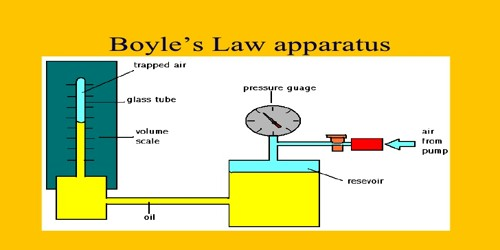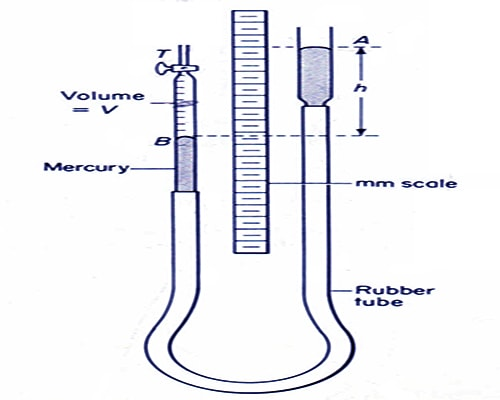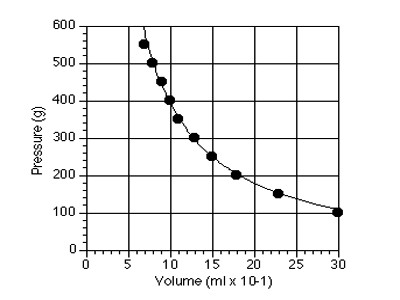Chemistry

# Verification of Boyle’s LawVerification of Boyle’s Law

Theory: Temperature remaining constant, the volume of a fixed mass of a gas is inversely proportional to the pressure on it.

If the volume and pressure of a fixed mass of gas are respectively V and P, then according to Boyle’s law-

V ∞ 1/P

or, PV = constant

Similarly, if the volumes of a fixed mass of gas at pressures P1, P2, P3 etc. are respectively V1, V2, V3 etc. then according to Boyle’s law we get, P1V1 = P2V2 = P3V3 = constant = K.

Apparatus: (1) Boyle’s instrument, (2) Barometer and (3) Thermometer.

Procedure: This experiment is performed in three stages, viz—

(A) at atmospheric pressure,

(B) at pressure greater than atmospheric pressure and

(C) at pressure less than atmospheric pressure.

(1) At the beginning of the experiment the atmospheric pressure is measured by barometer. Suppose it is P1.

(A) At atmospheric pressure

(2) By raising and lowering the tube-B it is kept at such a height so that mercury level in both the tubes remains at the same plane. In this stage, air confined in tube-A is equal to the atmospheric pressure.

(3) Readings are taken of the closed mouth of the tube-A and mercury level of the tube-A from the scale. From the difference of these two readings the column of confined air is found out. As the tube-A is of uniform diameter column of confined air is proportional to its volume. Product of the pressure of atmosphere and the length of air-column is found out.Figure: Verification of Boyle’s Law

(B) At pressure greater than atmospheric pressure:

(4) Now the tube-B is raised slowly. In this situation mercury column of the tube-B will be above the column of that in tube-A and air pressure of the confined air will be greater than atmospheric pressure. Reading is taken of both the mercury column and their difference is found out. By adding this difference with the atmospheric pressure air pressure of the confined air in the tube-A is found out. If P1 is the atmospheric pressure and h is the difference in mercury column of the two tubes, then pressure of the confined air is P = P1 + h. From the mercury level in the tube AB and the reading of the closed end, volume of the confined air is found out. By raising this tube-B progressively readings are taken for 5-6 times and in each time product of pressure and volume of the confined air is found out.

(C) At pressure lower than atmospheric pressure:

(5) Now the tube-B is lowered and it is kept at a place so that mercury column in tube-A remains above the mercury column in tube-B. In this situation, air pressure of the confined air in tube-A is lower than atmospheric pressure. Readings of the mercury columns of both the tubes are taken end their difference is determined. This difference is subtracted from the atmospheric pressure and the pressure of confined air in tube-A is determined. If P1 is the pressure of the atmosphere and h is the difference of the mercury columns, pressure of the confined air is, P = P1 – h. From the mercury level of the tube AB and reading of the closed end volume of the confined air is determined. By lowering progressively the tube-B readings are taken for 5-6 times and each time product of pressure and volume are found out.

(6) At the beginning and at the end pressures of the barometer are taken and average value is taken. Let it be P1.

Result:

Since P x V = constant, so the relation between P and V can be expressed by a graph. Now placing P along X-axis and V along Y-axis, if a graph is drawn it will be rectangular hyperbola which will prove that P x V = constant. But if a graph is drawn by P versus 1/V, it will be a straight line. This also proves that P x V = constant.Boyle’s Law is proved.

Precautions:

(1) Both the tubes should be completely vertical.

(2) Readings should be taken avoiding parallax.

(3) In order to maintain the temperature constant, the tube-B is to be raised or lowered very slowly.

(4) After taking reading each time one should wait for some time.

Discussion: If the cross-sections of the closed and open tubes are not uniform, then there will be an error in the result.